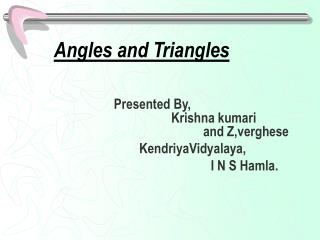DownloadDownload PresentationAngles and Triangles

# Angles and Triangles

Download Presentation## Angles and Triangles

- - - - - - - - - - - - - - - - - - - - - - - - - - - E N D - - - - - - - - - - - - - - - - - - - - - - - - - - -
##### Presentation Transcript

1. Angles and Triangles Presented By, Krishna kumari and Z,verghese KendriyaVidyalaya, I N S Hamla.

2. WE CAN DRAW A LINE SEGMENT ON APAPER AND IT HASDEFINITE LENGTHA RAY AND LINE HAVE NO DEFINITE LENGTH B A

3. TWO RAYS AND LINE SEGMENTS WITH THE COMMON END POINT FORM AN ANGLE A WHERE TWO END POINTS MEET IS CALLED VERTEX AND LINE SEGMENTS ARE CALLED ARMS A

4. ACUTE ANGLE A B C

5. RIGHT ANGLE A B C

6. OBTUSE ANGLE A C B

7. Triangles-Introduction • Definition: A plane figure bound by three lines in a plane is called a triangle.a triangle has 6 parts.(3sides & 3angles). • Here,AB,AC&BC are the sides,A,B & C are points. A C B

8. Triangle Definition:A triangle, HAS THREE LINE SEGM ENTS ,THR EE VERTIC E S AN D THR EE ANGLES A C B

9. Types Of Triangles On the basis of sides: 1. Scalene triangles 2. Isosceles triangles 3. Equilateral triangles. On the basis of angles: 4.Acute triangles 5.Right triangles 6.Obtuse triangles. An equilateral triangle is an isosceles triangle but the converse is not true.

10. Scalene Triangles • Definition:A triangle,no two of whose sides are equal, is called a scalene triangle.E.g.. C A B

11. Isosceles Triangle Definition:A triangle, two of whose sides are equal, is called an isosceles triangle.e.g. A C B

12. Equilateral Triangle • Definition: a triangle whose all sides are equal, is called an equilateral triangle.e.g. A B C

13. Acute triangle • Definition:A triangle each of whose angles is acute is called an acute angled triangle or simply acute triangle.e.g. A B C

14. Right Triangle • Definition:A triangle with one angle a right angle is called a right angled triangle or simply right triangle.e.g. A C B

15. Obtuse triangle • Definition:A triangle with one angle an obtuse angle is known as obtuse angled triangle or simply obtuse triangle.e.g. A C B

16. Triangle properties-1 A • Property 1:the sum of the three angles in a triangle is 180 degrees. • Property 2:if a side of a triangle is produced then the exterior angle so formed is equal to the sum of the two interior opposite angles. Here, angle A+angle B+angle C=180 degrees. C B A Here,angle ACD = angle A+angle B B C D